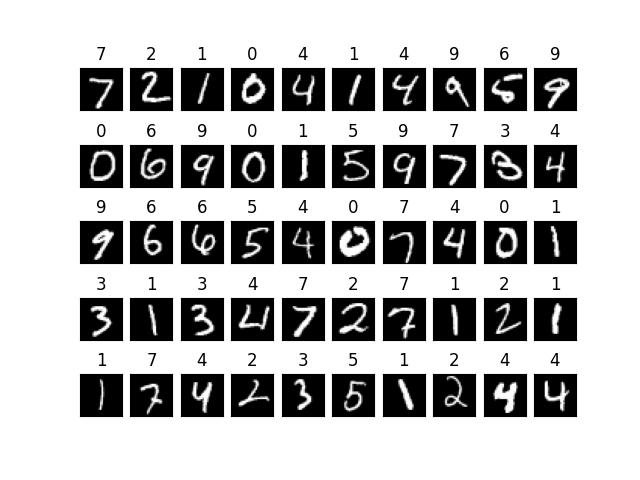# 支持向量機SVM

在〈支持向量機SVM〉中留言功能已關閉

# Support Vector Machines, SVM

SVM是一種非常經典的資料分類演算法，稱為支持向量機。其目的是在找出一個超平面，將二個不同的集合分開。

SVM分為線性可分集合及非線性可分集合。

# 線性可分集合

SVM模型將實例表示為空間中的點，這樣對應就使得單獨類別的實例被盡可能寬度間隔分開。然後將新的實例對映到同一空間，並基於它們落在間隔的哪一側來預測所屬類別。所以SVM簡單的說，就是計算中間灰色虛線的演算法。

# 手寫數字實例練訓

svm.SVC(C=100., kernel=’linear’)，C為懲罰係數，數字愈大，愈不能容忍誤差，當然也會降低速度。

from sklearn import datasets
from sklearn.model_selection import train_test_split
from sklearn.preprocessing import scale
import pylab as plt
from sklearn import svm
#載入資料並進行標準常態分配
data = scale(digits.data)

#拆開資料，3/4為訓練資料，1/4為預測資料
x_train, x_test, y_train, y_test = train_test_split(data, digits.target, train_size=0.75, random_state=42)

#建立模型
model = svm.SVC(C=100., kernel='linear')

#開始訓練模型
model.fit(x_train, y_train)

#傳入預測資料並取得結果
predict = model.predict(x_test)

for i in range(50):
sub=plt.subplot(5,10,i+1)
sub.set_title(predict[i])
sub.set_xticks([])
sub.set_yticks([])
sub.imshow(x_test[i].reshape(8,8), cmap='gray')
plt.show()# 使用 mnist 資料

model = svm.LinearSVC(C=100.)。LinearSVC也是線性演算法，但跟SVC不一樣，速度較快。

from keras.datasets import mnist
import pylab as plt
from sklearn import svm
x_train=x_train.reshape(60000,784)
x_train=x_train[:10000]
y_train=y_train[:10000]
x_test=x_test.reshape(10000,784)
x_test=x_test[:100]
y_test=y_test[:100]
#建立模型
model = svm.SVC(C=100., kernel='linear')#LinearSVC為線性演算，但演算法跟 SVC不一樣，速度較快，可用來訓練60000筆資料#model = svm.LinearSVC(C=100.)
#開始訓練模型
model.fit(x_train, y_train)

#儲存模型可以使用底下的二種方法
#joblib.dump(model, "mnist_model")
pickle.dump(model, open("model.pkl", "wb"), protocol=0)

#傳入預測資料並取得結果
predict = model.predict(x_test)
for i in range(50):
sub=plt.subplot(5,10,i+1)
sub.set_title(predict[i])
sub.set_xticks([])
sub.set_yticks([])
sub.imshow(x_test[i].reshape(28,28), cmap='gray')
plt.show()# 載入模型

import pickle
import joblib
from keras.datasets import mnist
import pylab as plt

with open("model.pkl",'rb') as f: